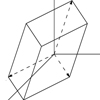18.06SC | Fall 2011 | Undergraduate

# Linear Algebra

Unit II: Least Squares, Determinants and Eigenvalues

## Cramer's Rule, Inverse Matrix and Volume

« Previous | Next »

### Session OverviewNow we start to use the determinant. Understanding the cofactor formula allows us to show that A-1 = (1/detA)CT, where C is the matrix of cofactors of A. Combining this formula with the equation x = A-1b gives us Cramer’s rule for solving Ax = b. Also, the absolute value of the determinant gives the volume of a box.

### Session Activities

#### Lecture Video and Summary

• Read Section 5.3 in the 4th or 5th edition.

### Check Yourself

#### Problems and Solutions

« Previous | Next »

## Course Info

Fall 2011
##### Learning Resource Types
Lecture Videos
Exams with Solutions
Lecture Notes
Recitation Videos
Problem Sets with Solutions
Simulations
Course Introduction
Instructor Insights